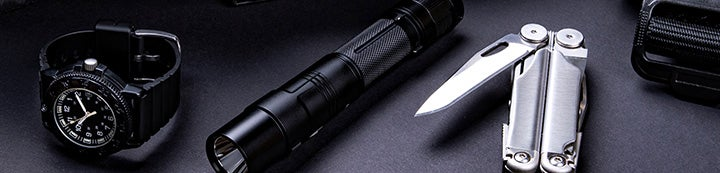1 - 3 of 3 Posts

roy

·
Registered
Joined
·
966 Posts
Discussion Starter · ·
The military uses something called a one time pad for very secure communications. I is unbreakable even with super-computers. It is also slow a tedious.

Here is how it works. A computer generates a number of random five alphabetic character groups on a pad of paper in two identical copies. The person going to send a message gets one copy. The person receiving the message gets the other. Your clear text message is written above the characters of the one time pad, skipping the first five character of the one time pad. Now for the tricky part. Each alphabetic character is assigned a numberic value from 1 to 26, A = 1, Z = 26. Add the values of the clear text character and the one time pad together. If the clear text letter is B, B = 2: and the one time pad character is M, M = 13; 2 + 13 = 15; 15 = O; O is what you send. If the number adds to more than 26 subtract 26. If the clear text letter is M; M = 13; and the one time pad character is O; O = 15; 13 + 15 = 28; 28 - 26 = 2; 2 = B. Send B.

To decode write the encoded message on the identical page of the one time pad. How do you know what page? The first 5 character group of the page is always sent in the clear. Flip through you pages untill you find the matching group. Starting on the second 5 character group write out the message above the characters of the one time pad. Do the same operation as mentioned above but this time with subtraction If the character receivd was O; O = 15; and the one time character is M; M = 13; 15 - 13 = 2. The decrypted letter is B. If you subtract and get a negative number add 26. If the encryped character is B and the one time character is O; 2 - 15 = -13; -13 + 26 = 13; 13 = M.

To get random number go here: RANDOM.ORG - Integer Generator. Put in 1 - 26, columns of 5.

You can get nearly the same level of security by using the pages of identical books. Send the page number in the clear as the first group.

I was an MI puke in another life.

•AquaHull

AquaHull

·
Registered
Joined
·
8,037 Posts
I've sent copies of books to a few friends.

•Nathan Jefferson

·